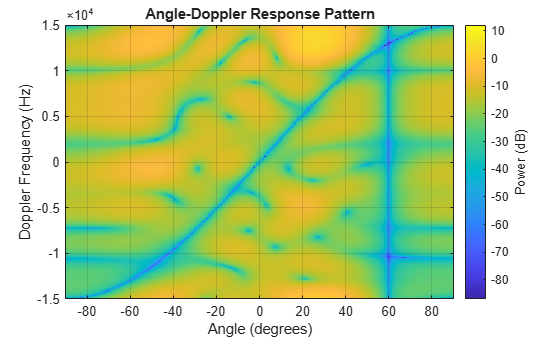# step

System object: phased.STAPSMIBeamformer
Package: phased

Perform SMI STAP processing on input data

## Syntax

```Y = step(H,X,CUTIDX) Y = step(H,X,CUTIDX,PRF) Y = step(H,X,CUTIDX,ANG) Y = step(H,X,CUTIDX,DOP) [Y,W] = step(___) ```

## Description

Note

Starting in R2016b, instead of using the `step` method to perform the operation defined by the System object™, you can call the object with arguments, as if it were a function. For example, ```y = step(obj,x)``` and `y = obj(x)` perform equivalent operations.

`Y = step(H,X,CUTIDX)` applies SMI processing to the input data, `X`. `X` must be a 3-dimensional M-by-N-by-P numeric array whose dimensions are (range, channels, pulses). The processing weights are calculated according to the range cell specified by `CUTIDX`. The targeting direction and the targeting Doppler are specified by `Direction` and `Doppler` properties, respectively. `Y` is a column vector of length M. This syntax is available when the `DirectionSource` property is `'Property'` and the `DopplerSource` property is `'Property'`.

The size of the first dimension of the input matrix can vary to simulate a changing signal length. A size change can occur, for example, in the case of a pulse waveform with variable pulse repetition frequency.

`Y = step(H,X,CUTIDX,PRF)` uses `PRF` as the pulse repetition frequency. This syntax is available when the `PRFSource` property is `'Input port'`.

`Y = step(H,X,CUTIDX,ANG)` uses `ANG` as the targeting direction. This syntax is available when the `DirectionSource` property is `'Input port'`. `ANG` must be a 2-by-1 vector in the form of `[AzimuthAngle; ElevationAngle]` (in degrees). The azimuth angle must be between –180 and 180. The elevation angle must be between –90 and 90.

`Y = step(H,X,CUTIDX,DOP)` uses `DOP` as the targeting Doppler frequency (in hertz). This syntax is available when the `DopplerSource` property is ```'Input port'```. `DOP` must be a scalar.

You can combine optional input arguments when their enabling properties are set: `Y = step(H,X,CUTIDX,ANG,DOP)`

`[Y,W] = step(___)` returns the additional output, `W`, as the processing weights. This syntax is available when the `WeightsOutputPort` property is `true`. `W` is a column vector of length N*P.

Note

The object performs an initialization the first time the object is executed. This initialization locks nontunable properties and input specifications, such as dimensions, complexity, and data type of the input data. If you change a nontunable property or an input specification, the System object issues an error. To change nontunable properties or inputs, you must first call the `release` method to unlock the object.

## Examples

expand all

Process a data cube using an SMI beamformer. The weights are calculated for the 71st cell of a collected data cube pointing in the azimuth and elevation direction (45°,-35°) and with a Doppler of 12.980 kHz.

Load the cube data and create the SMI beamformer.

```load STAPExampleData; beamformer = phased.STAPSMIBeamformer('SensorArray',STAPEx_HArray, ... 'PRF',STAPEx_PRF,'PropagationSpeed',STAPEx_PropagationSpeed, ... 'OperatingFrequency',STAPEx_OperatingFrequency, ... 'NumTrainingCells',100,'WeightsOutputPort',true, ... 'DirectionSource','Input port','DopplerSource','Input port'); [y,w] = beamformer(STAPEx_ReceivePulse,71,[45;-35],12.980e3);```

Plot the angle-doppler response.

```response = phased.AngleDopplerResponse( ... 'SensorArray',beamformer.SensorArray, ... 'OperatingFrequency',beamformer.OperatingFrequency, ... 'PRF',beamformer.PRF,'PropagationSpeed',beamformer.PropagationSpeed); plotResponse(response,w)```